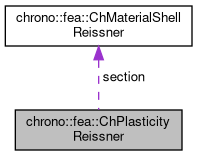chrono::fea::ChPlasticityReissner Class Referenceabstract

## Description

Base interface for plasticity of 6-field Reissner-Mindlin shells (kinematically-exact shell theory as in Witkowski et al.) to be used in a ChMaterialShellReissner.

Children classes must implement the ComputeStressWithReturnMapping to compute effective stress and strain given a tentative strain that might violate the yeld function. Inherited materials do not define any thickness, which should be a property of the element or its layer(s) using this material.

#include <ChMaterialShellReissner.h>

Collaboration diagram for chrono::fea::ChPlasticityReissner:[legend]

## Public Member Functions

virtual bool ComputeStressWithReturnMapping (ChVector<> &n_u, ChVector<> &n_v, ChVector<> &m_u, ChVector<> &m_v, ChShellReissnerInternalData &data_new, const ChVector<> &eps_u_trial, const ChVector<> &eps_v_trial, const ChVector<> &kur_u_trial, const ChVector<> &kur_v_trial, const ChShellReissnerInternalData &data, const double z_inf, const double z_sup, const double angle)=0
Given a trial strain, it computes the effective stress and strain by clamping against the yeld surface. More...

virtual void ComputeStiffnessMatrixElastoplastic (ChMatrixRef K, const ChVector<> &eps_u, const ChVector<> &eps_v, const ChVector<> &kur_u, const ChVector<> &kur_v, const ChShellReissnerInternalData &data, const double z_inf, const double z_sup, const double angle)
Compute the 12x12 tangent material stiffness matrix [Km] = dσ/dε, given actual internal data and deformation and curvature (if needed). More...

virtual void CreatePlasticityData (int numpoints, std::vector< std::unique_ptr< ChShellReissnerInternalData >> &plastic_data)

## Public Attributes

ChMaterialShellReissnersection

double nr_yeld_tolerance

int nr_yeld_maxiters

## ◆ ComputeStiffnessMatrixElastoplastic()

 void chrono::fea::ChPlasticityReissner::ComputeStiffnessMatrixElastoplastic ( ChMatrixRef K, const ChVector<> & eps_u, const ChVector<> & eps_v, const ChVector<> & kur_u, const ChVector<> & kur_v, const ChShellReissnerInternalData & data, const double z_inf, const double z_sup, const double angle )
virtual

Compute the 12x12 tangent material stiffness matrix [Km] = dσ/dε, given actual internal data and deformation and curvature (if needed).

If in plastic regime, uses elastoplastic matrix, otherwise uses elastic. This must be overridden by subclasses if an analytical solution is known (preferred for high performance), otherwise the base behaviour here is to compute [Km] by numerical differentiation calling ComputeStressWithReturnMapping() multiple times.

Parameters
 K 12x12 material elastoplastic stiffness matrix values here eps_u strains along u direction eps_v strains along v direction kur_u curvature along u direction kur_v curvature along v direction data updated material internal variables, at this point including {p_strain_e, p_strain_k, p_strain_acc} z_inf layer lower z value (along thickness coord) z_sup layer upper z value (along thickness coord) angle layer angle respect to x (if needed)

## ◆ ComputeStressWithReturnMapping()

 virtual bool chrono::fea::ChPlasticityReissner::ComputeStressWithReturnMapping ( ChVector<> & n_u, ChVector<> & n_v, ChVector<> & m_u, ChVector<> & m_v, ChShellReissnerInternalData & data_new, const ChVector<> & eps_u_trial, const ChVector<> & eps_v_trial, const ChVector<> & kur_u_trial, const ChVector<> & kur_v_trial, const ChShellReissnerInternalData & data, const double z_inf, const double z_sup, const double angle )
pure virtual

Given a trial strain, it computes the effective stress and strain by clamping against the yeld surface.

An implicit return mapping integration step is computed automatically per each call of this function. Note: for the elastic part, it must use the elasticity model in this->section->elasticity. If not beyond yeld, simply: elastic strain = tot strain - plastic strain If it is beyond yeld: elastic strain is computed by fully implicit strain integration with return mapping, and plastic strains in "data_new" are updated. Returns true if it had to do return mapping, false if it was in elastic regime This MUST be implemented by subclasses.

Parameters
 n_u forces along u direction (per unit length) n_v forces along v direction (per unit length) m_u torques along u direction (per unit length) m_v torques along v direction (per unit length) data_new updated material internal variables, at this point, including {p_strain_e, p_strain_k, p_strain_acc} eps_u_trial trial strains along u direction eps_v_trial trial strains along v direction kur_u_trial trial curvature along u direction kur_v_trial trial curvature along v direction data trial material internal variables, at this point, including {p_strain_e, p_strain_k, p_strain_acc} z_inf layer lower z value (along thickness coord) z_sup layer upper z value (along thickness coord) angle layer angle respect to x (if needed)

The documentation for this class was generated from the following files:
• /builds/uwsbel/chrono/src/chrono/fea/ChMaterialShellReissner.h
• /builds/uwsbel/chrono/src/chrono/fea/ChMaterialShellReissner.cpp